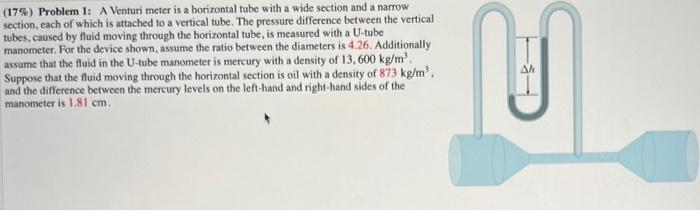# Question Solved1 Answer (17\%) Problem 1: A Venturi meter is a horizontal tube with a wide section and a narrow section, each of which is attached to a vertical tube. The pressure difference between the vertical tubes, caused by fluid moving through the horizontal tube, is measured with a U-tube manometer. For the device shown, assume the ratio between the diameters is $$4.26$$. (17\%) Problem 1: A Venturi meter is a horizontal tube with a wide section and a narrow section, each of which is attached to a vertical tube. The pressure difference between the vertical tubes, caused by fluid moving through the horizontal tube, is measured with a U-tube manometer. For the device shown, assume the ratio between the diameters is 4.26. Additionally assume that the fluid in the U-tube manometer is mercury with a density of 13,600 kg/m3. Suppose that the fluid moving through the horizontal section is oil with a density of 873 kg/m3, and the difference between the mereury levels on the left-hand and right-hand sides of the manometer is 1.81 cm.Transcribed Image Text: (17\%) Problem 1: A Venturi meter is a horizontal tube with a wide section and a narrow section, each of which is attached to a vertical tube. The pressure difference between the vertical tubes, caused by fluid moving through the horizontal tube, is measured with a U-tube manometer. For the device shown, assume the ratio between the diameters is 4.26. Additionally assume that the fluid in the U-tube manometer is mercury with a density of 13,600 kg/m3. Suppose that the fluid moving through the horizontal section is oil with a density of 873 kg/m3, and the difference between the mereury levels on the left-hand and right-hand sides of the manometer is 1.81 cm.
More
Transcribed Image Text: (17\%) Problem 1: A Venturi meter is a horizontal tube with a wide section and a narrow section, each of which is attached to a vertical tube. The pressure difference between the vertical tubes, caused by fluid moving through the horizontal tube, is measured with a U-tube manometer. For the device shown, assume the ratio between the diameters is 4.26. Additionally assume that the fluid in the U-tube manometer is mercury with a density of 13,600 kg/m3. Suppose that the fluid moving through the horizontal section is oil with a density of 873 kg/m3, and the difference between the mereury levels on the left-hand and right-hand sides of the manometer is 1.81 cm.